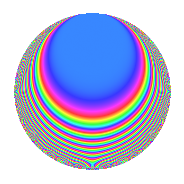# Properties

 Label 2004.1.g.eLevel 2004 Weight 1 Character orbit 2004.g Analytic conductor 1.000 Analytic rank 0 Dimension 10 Projective image $$D_{22}$$ CM disc. -167 Inner twists 4

# Related objects

## Newspace parameters

 Level: $$N$$ = $$2004 = 2^{2} \cdot 3 \cdot 167$$ Weight: $$k$$ = $$1$$ Character orbit: $$[\chi]$$ = 2004.g (of order $$2$$ and degree $$1$$)

## Newform invariants

 Self dual: No Analytic conductor: $$1.00012628532$$ Analytic rank: $$0$$ Dimension: $$10$$ Coefficient field: $$\Q(\zeta_{22})$$ Coefficient ring: $$\Z[a_1, a_2]$$ Coefficient ring index: $$1$$ Projective image $$D_{22}$$ Projective field Galois closure of $$\mathbb{Q}[x]/(x^{22} + \cdots)$$

## $q$-expansion

The $$q$$-expansion and trace form are shown below.

 $$f(q)$$ $$=$$ $$q$$ $$+ \zeta_{22}^{6} q^{2}$$ $$+ \zeta_{22}^{2} q^{3}$$ $$-\zeta_{22} q^{4}$$ $$+ \zeta_{22}^{8} q^{6}$$ $$+ ( \zeta_{22}^{3} + \zeta_{22}^{8} ) q^{7}$$ $$-\zeta_{22}^{7} q^{8}$$ $$+ \zeta_{22}^{4} q^{9}$$ $$+O(q^{10})$$ $$q$$ $$+ \zeta_{22}^{6} q^{2}$$ $$+ \zeta_{22}^{2} q^{3}$$ $$-\zeta_{22} q^{4}$$ $$+ \zeta_{22}^{8} q^{6}$$ $$+ ( \zeta_{22}^{3} + \zeta_{22}^{8} ) q^{7}$$ $$-\zeta_{22}^{7} q^{8}$$ $$+ \zeta_{22}^{4} q^{9}$$ $$+ ( \zeta_{22} - \zeta_{22}^{10} ) q^{11}$$ $$-\zeta_{22}^{3} q^{12}$$ $$+ ( -\zeta_{22}^{3} + \zeta_{22}^{9} ) q^{14}$$ $$+ \zeta_{22}^{2} q^{16}$$ $$+ \zeta_{22}^{10} q^{18}$$ $$+ ( -\zeta_{22}^{4} - \zeta_{22}^{7} ) q^{19}$$ $$+ ( \zeta_{22}^{5} + \zeta_{22}^{10} ) q^{21}$$ $$+ ( \zeta_{22}^{5} + \zeta_{22}^{7} ) q^{22}$$ $$-\zeta_{22}^{9} q^{24}$$ $$- q^{25}$$ $$+ \zeta_{22}^{6} q^{27}$$ $$+ ( -\zeta_{22}^{4} - \zeta_{22}^{9} ) q^{28}$$ $$+ ( \zeta_{22}^{3} + \zeta_{22}^{8} ) q^{29}$$ $$+ ( -\zeta_{22}^{5} - \zeta_{22}^{6} ) q^{31}$$ $$+ \zeta_{22}^{8} q^{32}$$ $$+ ( \zeta_{22} + \zeta_{22}^{3} ) q^{33}$$ $$-\zeta_{22}^{5} q^{36}$$ $$+ ( \zeta_{22}^{2} - \zeta_{22}^{10} ) q^{38}$$ $$+ ( -1 - \zeta_{22}^{5} ) q^{42}$$ $$+ ( -1 - \zeta_{22}^{2} ) q^{44}$$ $$+ ( -\zeta_{22}^{4} + \zeta_{22}^{7} ) q^{47}$$ $$+ \zeta_{22}^{4} q^{48}$$ $$+ ( -1 - \zeta_{22}^{5} + \zeta_{22}^{6} ) q^{49}$$ $$-\zeta_{22}^{6} q^{50}$$ $$-\zeta_{22} q^{54}$$ $$+ ( \zeta_{22}^{4} - \zeta_{22}^{10} ) q^{56}$$ $$+ ( -\zeta_{22}^{6} - \zeta_{22}^{9} ) q^{57}$$ $$+ ( -\zeta_{22}^{3} + \zeta_{22}^{9} ) q^{58}$$ $$+ ( -\zeta_{22}^{2} + \zeta_{22}^{9} ) q^{61}$$ $$+ ( 1 + \zeta_{22} ) q^{62}$$ $$+ ( -\zeta_{22} + \zeta_{22}^{7} ) q^{63}$$ $$-\zeta_{22}^{3} q^{64}$$ $$+ ( \zeta_{22}^{7} + \zeta_{22}^{9} ) q^{66}$$ $$+ q^{72}$$ $$-\zeta_{22}^{2} q^{75}$$ $$+ ( \zeta_{22}^{5} + \zeta_{22}^{8} ) q^{76}$$ $$+ ( \zeta_{22}^{2} + \zeta_{22}^{4} + \zeta_{22}^{7} + \zeta_{22}^{9} ) q^{77}$$ $$+ \zeta_{22}^{8} q^{81}$$ $$+ ( 1 - \zeta_{22}^{6} ) q^{84}$$ $$+ ( \zeta_{22}^{5} + \zeta_{22}^{10} ) q^{87}$$ $$+ ( -\zeta_{22}^{6} - \zeta_{22}^{8} ) q^{88}$$ $$+ ( -\zeta_{22}^{2} - \zeta_{22}^{9} ) q^{89}$$ $$+ ( -\zeta_{22}^{7} - \zeta_{22}^{8} ) q^{93}$$ $$+ ( -\zeta_{22}^{2} - \zeta_{22}^{10} ) q^{94}$$ $$+ \zeta_{22}^{10} q^{96}$$ $$+ ( -\zeta_{22} + \zeta_{22}^{10} ) q^{97}$$ $$+ ( 1 - \zeta_{22} - \zeta_{22}^{6} ) q^{98}$$ $$+ ( \zeta_{22}^{3} + \zeta_{22}^{5} ) q^{99}$$ $$+O(q^{100})$$ $$\operatorname{Tr}(f)(q)$$ $$=$$ $$10q$$ $$\mathstrut -\mathstrut q^{2}$$ $$\mathstrut -\mathstrut q^{3}$$ $$\mathstrut -\mathstrut q^{4}$$ $$\mathstrut -\mathstrut q^{6}$$ $$\mathstrut -\mathstrut q^{8}$$ $$\mathstrut -\mathstrut q^{9}$$ $$\mathstrut +\mathstrut O(q^{10})$$ $$10q$$ $$\mathstrut -\mathstrut q^{2}$$ $$\mathstrut -\mathstrut q^{3}$$ $$\mathstrut -\mathstrut q^{4}$$ $$\mathstrut -\mathstrut q^{6}$$ $$\mathstrut -\mathstrut q^{8}$$ $$\mathstrut -\mathstrut q^{9}$$ $$\mathstrut +\mathstrut 2q^{11}$$ $$\mathstrut -\mathstrut q^{12}$$ $$\mathstrut -\mathstrut q^{16}$$ $$\mathstrut -\mathstrut q^{18}$$ $$\mathstrut +\mathstrut 2q^{22}$$ $$\mathstrut -\mathstrut q^{24}$$ $$\mathstrut -\mathstrut 10q^{25}$$ $$\mathstrut -\mathstrut q^{27}$$ $$\mathstrut -\mathstrut q^{32}$$ $$\mathstrut +\mathstrut 2q^{33}$$ $$\mathstrut -\mathstrut q^{36}$$ $$\mathstrut -\mathstrut 11q^{42}$$ $$\mathstrut -\mathstrut 9q^{44}$$ $$\mathstrut +\mathstrut 2q^{47}$$ $$\mathstrut -\mathstrut q^{48}$$ $$\mathstrut -\mathstrut 12q^{49}$$ $$\mathstrut +\mathstrut q^{50}$$ $$\mathstrut -\mathstrut q^{54}$$ $$\mathstrut +\mathstrut 2q^{61}$$ $$\mathstrut +\mathstrut 11q^{62}$$ $$\mathstrut -\mathstrut q^{64}$$ $$\mathstrut +\mathstrut 2q^{66}$$ $$\mathstrut +\mathstrut 10q^{72}$$ $$\mathstrut +\mathstrut q^{75}$$ $$\mathstrut -\mathstrut q^{81}$$ $$\mathstrut +\mathstrut 11q^{84}$$ $$\mathstrut +\mathstrut 2q^{88}$$ $$\mathstrut +\mathstrut 2q^{94}$$ $$\mathstrut -\mathstrut q^{96}$$ $$\mathstrut -\mathstrut 2q^{97}$$ $$\mathstrut +\mathstrut 10q^{98}$$ $$\mathstrut +\mathstrut 2q^{99}$$ $$\mathstrut +\mathstrut O(q^{100})$$

## Character Values

We give the values of $$\chi$$ on generators for $$\left(\mathbb{Z}/2004\mathbb{Z}\right)^\times$$.

 $$n$$ $$673$$ $$1003$$ $$1337$$ $$\chi(n)$$ $$-1$$ $$-1$$ $$-1$$

## Embeddings

For each embedding $$\iota_m$$ of the coefficient field, the values $$\iota_m(a_n)$$ are shown below.

For more information on an embedded modular form you can click on its label.

Label $$\iota_m(\nu)$$ $$a_{2}$$ $$a_{3}$$ $$a_{4}$$ $$a_{5}$$ $$a_{6}$$ $$a_{7}$$ $$a_{8}$$ $$a_{9}$$ $$a_{10}$$
2003.1
 −0.841254 − 0.540641i −0.841254 + 0.540641i 0.142315 − 0.989821i 0.142315 + 0.989821i 0.959493 − 0.281733i 0.959493 + 0.281733i 0.654861 + 0.755750i 0.654861 − 0.755750i −0.415415 + 0.909632i −0.415415 − 0.909632i
−0.959493 0.281733i 0.415415 + 0.909632i 0.841254 + 0.540641i 0 −0.142315 0.989821i 1.97964i −0.654861 0.755750i −0.654861 + 0.755750i 0
2003.2 −0.959493 + 0.281733i 0.415415 0.909632i 0.841254 0.540641i 0 −0.142315 + 0.989821i 1.97964i −0.654861 + 0.755750i −0.654861 0.755750i 0
2003.3 −0.654861 0.755750i −0.959493 0.281733i −0.142315 + 0.989821i 0 0.415415 + 0.909632i 1.81926i 0.841254 0.540641i 0.841254 + 0.540641i 0
2003.4 −0.654861 + 0.755750i −0.959493 + 0.281733i −0.142315 0.989821i 0 0.415415 0.909632i 1.81926i 0.841254 + 0.540641i 0.841254 0.540641i 0
2003.5 −0.142315 0.989821i 0.841254 0.540641i −0.959493 + 0.281733i 0 −0.654861 0.755750i 1.51150i 0.415415 + 0.909632i 0.415415 0.909632i 0
2003.6 −0.142315 + 0.989821i 0.841254 + 0.540641i −0.959493 0.281733i 0 −0.654861 + 0.755750i 1.51150i 0.415415 0.909632i 0.415415 + 0.909632i 0
2003.7 0.415415 0.909632i −0.142315 + 0.989821i −0.654861 0.755750i 0 0.841254 + 0.540641i 1.08128i −0.959493 + 0.281733i −0.959493 0.281733i 0
2003.8 0.415415 + 0.909632i −0.142315 0.989821i −0.654861 + 0.755750i 0 0.841254 0.540641i 1.08128i −0.959493 0.281733i −0.959493 + 0.281733i 0
2003.9 0.841254 0.540641i −0.654861 0.755750i 0.415415 0.909632i 0 −0.959493 0.281733i 0.563465i −0.142315 0.989821i −0.142315 + 0.989821i 0
2003.10 0.841254 + 0.540641i −0.654861 + 0.755750i 0.415415 + 0.909632i 0 −0.959493 + 0.281733i 0.563465i −0.142315 + 0.989821i −0.142315 0.989821i 0
 $$n$$: e.g. 2-40 or 990-1000 Embeddings: e.g. 1-3 or 2003.10 Significant digits: Format: Complex embeddings Normalized embeddings Satake parameters Satake angles

## Inner twists

Char. orbit Parity Mult. Self Twist Proved
1.a Even 1 trivial yes
167.b Odd 1 CM by $$\Q(\sqrt{-167})$$ yes
12.b Even 1 yes
2004.g Odd 1 yes

## Hecke kernels

This newform can be constructed as the intersection of the kernels of the following linear operators acting on $$S_{1}^{\mathrm{new}}(2004, [\chi])$$:

 $$T_{5}$$ $$T_{7}^{10}$$ $$\mathstrut +\mathstrut 11 T_{7}^{8}$$ $$\mathstrut +\mathstrut 44 T_{7}^{6}$$ $$\mathstrut +\mathstrut 77 T_{7}^{4}$$ $$\mathstrut +\mathstrut 55 T_{7}^{2}$$ $$\mathstrut +\mathstrut 11$$ $$T_{11}^{5}$$ $$\mathstrut -\mathstrut T_{11}^{4}$$ $$\mathstrut -\mathstrut 4 T_{11}^{3}$$ $$\mathstrut +\mathstrut 3 T_{11}^{2}$$ $$\mathstrut +\mathstrut 3 T_{11}$$ $$\mathstrut -\mathstrut 1$$ $$T_{179}^{5}$$ $$\mathstrut +\mathstrut T_{179}^{4}$$ $$\mathstrut -\mathstrut 4 T_{179}^{3}$$ $$\mathstrut -\mathstrut 3 T_{179}^{2}$$ $$\mathstrut +\mathstrut 3 T_{179}$$ $$\mathstrut +\mathstrut 1$$